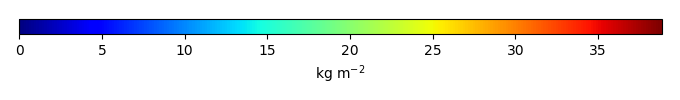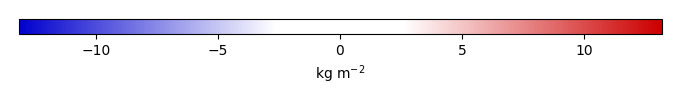# Mean State

Period Mean (original grids) [Pg]
Model Period Mean (intersection) [Pg]
Model Period Mean (complement) [Pg]
Benchmark Period Mean (intersection) [Pg]
Benchmark Period Mean (complement) [Pg]
Bias [kg m-2]
Bias Score 
Spatial Distribution Score 
Overall Score 
Benchmark [-] 26.2
CLM4 [-] 582. 21.9 560. 26.1 0.0878 -0.299 0.634 0.853 0.743
CLM4.5 [-] 560. 24.1 536. 26.1 0.0878 0.0184 0.615 0.873 0.744
CLM5 [-] 589. 21.7 567. 26.1 0.0878 -0.243 0.616 0.874 0.745
Period Mean (original grids) [Pg]
Model Period Mean (intersection) [Pg]
Model Period Mean (complement) [Pg]
Benchmark Period Mean (intersection) [Pg]
Benchmark Period Mean (complement) [Pg]
Bias [kg m-2]
Bias Score 
Spatial Distribution Score 
Overall Score 
Benchmark [-] 0.00338
CLM4 [-] 5.39 0.00986 5.08 0.00338 0.0933 0.616 0.679 0.647
CLM4.5 [-] 11.4 0.0180 11.1 0.00338 0.232 0.361 0.296 0.328
CLM5 [-] 11.9 0.0255 11.6 0.00338 0.302 0.360 0.401 0.380
Period Mean (original grids) [Pg]
Model Period Mean (intersection) [Pg]
Model Period Mean (complement) [Pg]
Benchmark Period Mean (intersection) [Pg]
Benchmark Period Mean (complement) [Pg]
Bias [kg m-2]
Bias Score 
Spatial Distribution Score 
Overall Score 
Benchmark [-] 17.5
CLM4 [-] 16.4 16.0 0.266 17.4 0.0333 -0.0421 0.666 0.908 0.787
CLM4.5 [-] 20.4 19.9 0.326 17.4 0.0333 0.973 0.680 0.896 0.788
CLM5 [-] 17.8 17.4 0.303 17.4 0.0333 0.386 0.671 0.907 0.789
Period Mean (original grids) [Pg]
Model Period Mean (intersection) [Pg]
Model Period Mean (complement) [Pg]
Benchmark Period Mean (intersection) [Pg]
Benchmark Period Mean (complement) [Pg]
Bias [kg m-2]
Bias Score 
Spatial Distribution Score 
Overall Score 
Benchmark [-] 0.222
CLM4 [-] 25.1 0.735 24.5 0.222 6.06 0.353 0.395 0.374
CLM4.5 [-] 34.8 0.804 34.2 0.222 6.81 0.333 0.460 0.396
CLM5 [-] 56.8 0.907 56.1 0.222 8.04 0.289 0.391 0.340
Period Mean (original grids) [Pg]
Model Period Mean (intersection) [Pg]
Model Period Mean (complement) [Pg]
Benchmark Period Mean (intersection) [Pg]
Benchmark Period Mean (complement) [Pg]
Bias [kg m-2]
Bias Score 
Spatial Distribution Score 
Overall Score 
Benchmark [-] 8.47
CLM4 [-] 5.11 5.12 0.0325 8.42 0.0520 -0.736 0.573 0.571 0.572
CLM4.5 [-] 3.42 3.42 0.0387 8.42 0.0520 -1.16 0.489 0.369 0.429
CLM5 [-] 3.49 3.46 0.0505 8.42 0.0520 -1.12 0.507 0.698 0.603

# Temporally integrated period mean

BENCHMARK MEANMODEL MEANBIASBIAS SCORESPATIAL TAYLOR DIAGRAMMODEL COLORS# Spatially integrated regional mean

MODEL COLORS# All Models

BenchmarkCLM4CLM4.5CLM5